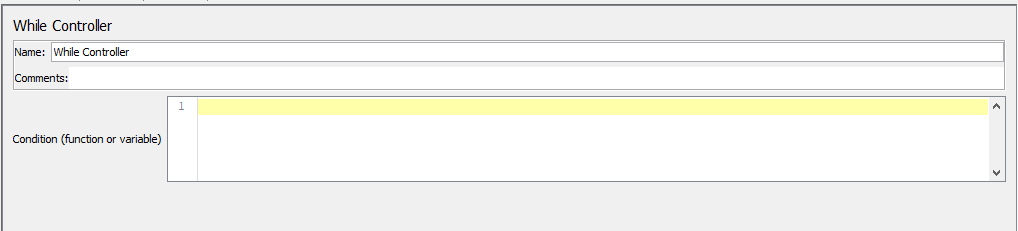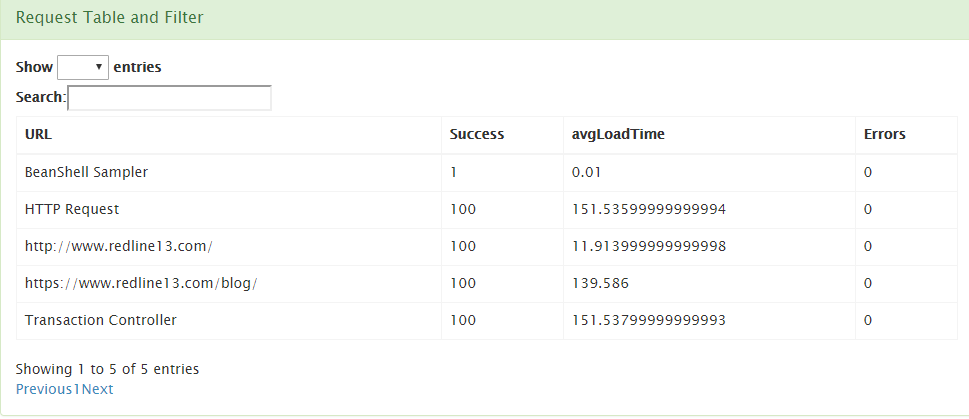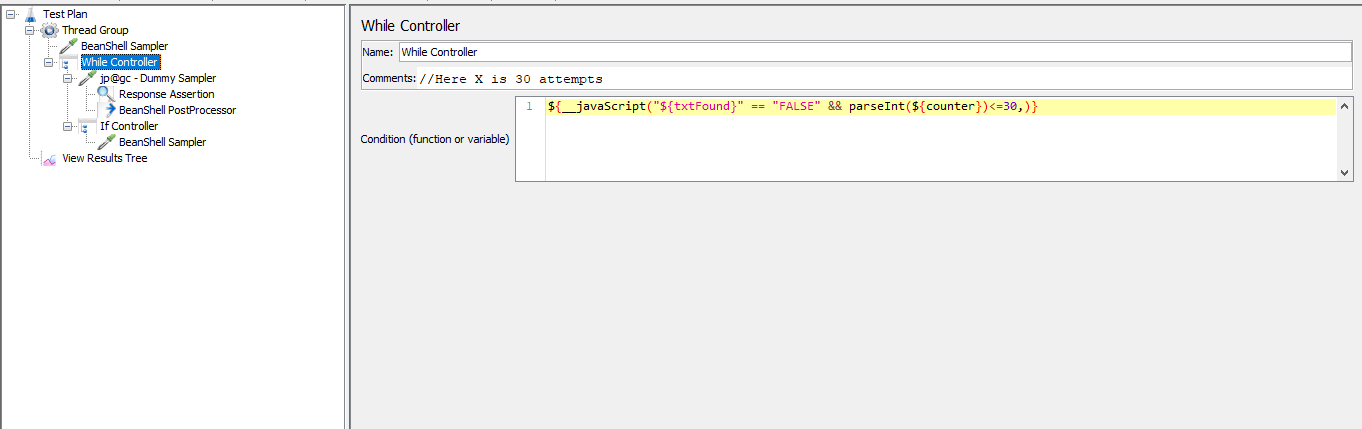A while loop is a control flow statement that allows code to be executed repeatedly based on a given Boolean condition. It’s just a repeated IF→THEN statement. IF the condition is true, THEN execute the statements inside the IF block. JMeter implements this while loop by using the JMeter While Controller.

The JMeter While Controller basically runs children Samplers and the controller continues to run until the condition becomes false in the condition field.

The possible condition values could be:

• blank – When the condition is blank , the While Controller will exit when the last sampler in the loop fails.
• LAST – Exit loop when last sample in loop fails. If the last sample just before the loop failed, don’t enter loop.
• JMeter Function or Variable – The samplers inside the While Controller will be run until a variable or function evaluation result is “true”.

The condition can be any variable or function that eventually evaluates to the string “false”. This allows the use of __jexl3, __groovy ,__javaScript function, properties or variables as needed.As you can see in the above screenshot, the condition should be a “function or variable”. The while controller expects the condition to be “true” or “false”.

Therefore, variable comparison like “\${Variable}”==”Value” does not work as the comparison does not evaluates to either “true” or “false”. To evaluate such variable comparisons to “true” or “false” use __Javascript function .\${__javaScript(“\${Variable}”==”Value”,)}, which evaluates to “true” or “false”. Also you can use the \${__jexl3(,)} function to evaluate the result.

## Some Common Use Cases

While with Counter: A while loop can be seen as a for loop when used in conjunction with a counter. You can download a sample JMX file from here.

This example illustrates how to use the Beanshell sampler JSR223 post processor combined with a While Controller to loop for a given number of iterations. The Thread group contains:

• Beanshell Sampler: Initializes the counter to the value 1 `.vars.put("counter","1");`
• While Controller: It checks for the counter value: `\${__javaScript(parseInt(vars.get("counter"))<=100)`

//100 is the loop count

• JSR223 post processor: It increments the counter:

`int counter = Integer.parseInt(vars.get("counter")) +1;`
`vars.put("counter",Integer.toString(counter));`

Here are the actual test results of this example:

###Since the loop count is 100, the http request executed 100 times. Here are detailed test results.

Execute until: Suppose you would like to perform a while loop using JMeter and you would like to quit the loop if the response assertion has been successful. Otherwise you would like to fail if it doesn’t respond correctly in X number of attempts.

The test plan could be something like:

Beanshell/JSR223 sampler to set text found flag and counter
`vars.put("counter","1");`

`vars.put("txtFound","FALSE")`

While Controller
Condition = `\${__javaScript("\${txtFound}" == "FALSE" && parseInt(\${counter})<=X,)}`

. . .
. . .

Beanshell/JSR223 post processor

`// To set counter value and text found flag`

`int counter = Integer.parseInt(vars.get("counter"));`

`if(counter==X)`

`vars.put("txtFound","TRUE");`

`counter++;`

`vars.put("counter",Integer.toString(counter));`

Response Assertion
`// Set your text assertion here`
`// This will results in \${JMeterThread.last_sample_ok} = TRUE if text found`
`IF Controller --FOUND`
Condition = `\${JMeterThread.last_sample_ok}`
Beanshell/JSR223 function //set-found-condition
`vars.put("txtFound","TRUE") // this will be inverted to FALSE in the next WHILE cycle's condition, WHILE cycle will be exited`You can download sample test plan from here and run the test as was done above. With RedLine13, you can run a JMeter Load Test with your JMX script of any mobile application, web application, or API.

Run your own test on RedLine13.